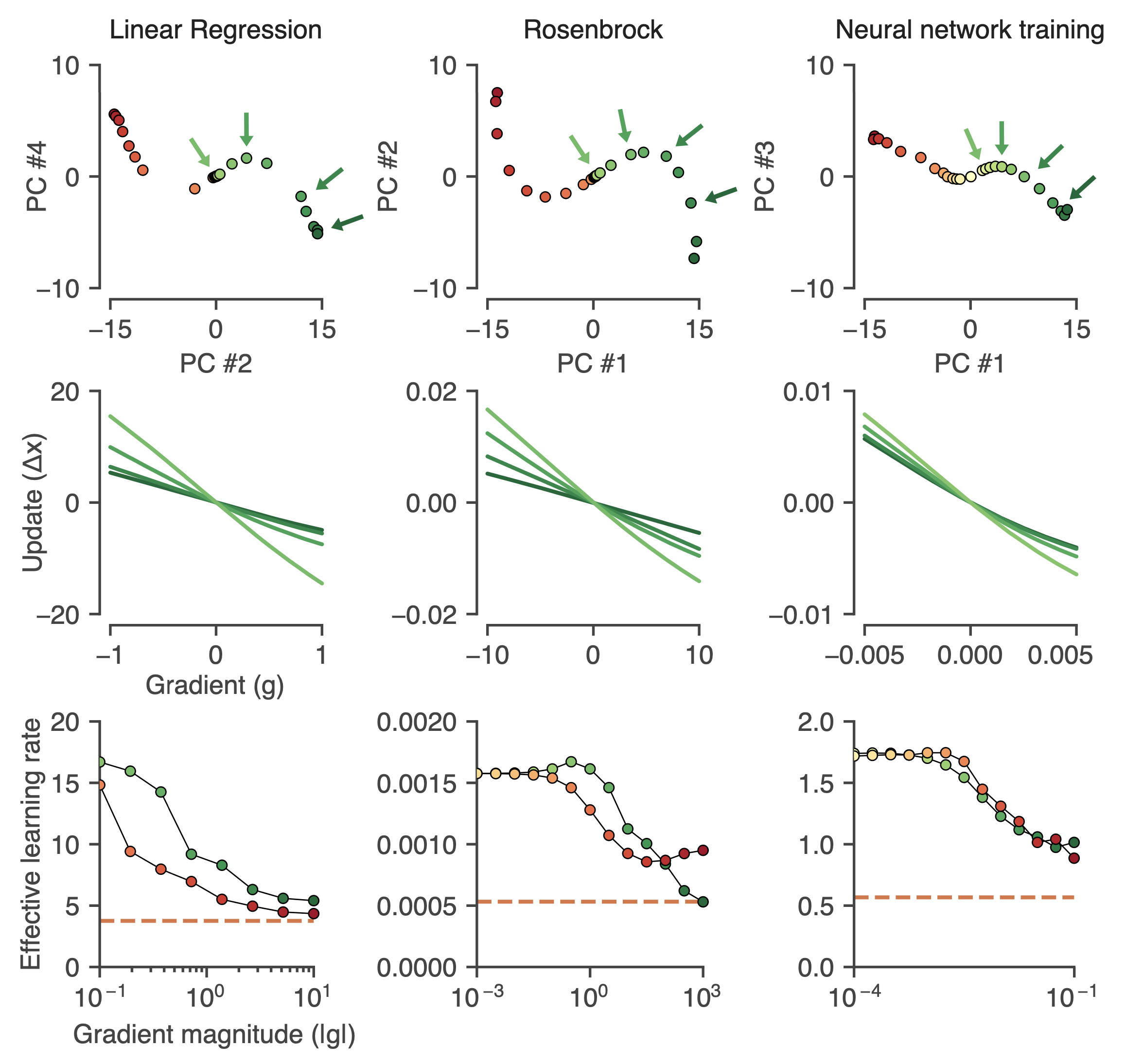# Why Learned Optimizers Outperform Standard Optimizers like Adam

##### Reverse engineering learned optimizers reveals known and novel mechanisms by Google BrainOptimizers, such as momentum (Polyak, 1964), AdaGrad (Duchi et al., 2011), RMSProp (Tieleman & Hinton, 2012), or Adam (Kingma & Ba, 2014), are algorithms underlying in nearly all machine learning. Combined with the loss function, they are the key pieces that enable machine learning to work. These algorithms use simple update rules derived from intuitive mechanisms and theoretical principles, a mathematical way of measuring how wrong your predictions are, and tune it to become better.

Recent research thread has focused on learning-based optimization algorithms; they called it learned optimizers. It has been shown that learned optimizers outperform “hand-designed” optimizers, like Adam, by directly parameterizing and training an optimizer on the distribution of tasks (Andrychowicz et al., 2016; Wichrowska et al., 2017; Lv et al., 2017; Bello et al., 2017; Li & Malik, 2016; Metz et al., 2019; 2020).

Despite the improvements in the performance from using these learned optimizers, researchers still lack an understanding of how it works. Researchers highlighted that understanding learned optimizers’ underlying mechanisms could improve robustness (Wichrowska et al., 2017; Lv et al., 2017), meta-training (Metz et al., 2019), and generalization (Metz et al., 2020) of learned optimizers. Identifying their operational flaws while also deepening our insight into why key machine learning mechanisms work and how to improve them.

For momentum and other standard optimizers, the state variables are low-dimensional, so their dynamics are straightforward, and it is simple to describe its behavior. But in contrast, learned optimizers have high-dimensional state variables, so these systems learn complex nonlinear dynamics, which is challenging to extract intuitive descriptions of a learned optimizer’s behavior.

Researchers have used common optimization techniques to tune optimizers over the years–techniques such as momentum, gradient clipping, learning rate schedules, and learning rate adaptation. These are intuitive mechanisms that have been shown to help minimize the loss function over parameters.

Are learned optimizers only learning a clever combination of known techniques? Or have they discovered fundamentally new behaviors that have not yet been proposed in the optimization literature?

This Google Brain research team attempts to shed some light on the matter. The team developed tools for isolating and elucidating mechanisms in nonlinear, high-dimensional learned optimization algorithms. This paper, Reverse engineering learned optimizers reveals known and novel mechanisms, is currently under review for ICLR 2021.

The researchers trained the learned optimizers on three tasks that are fast to train, which is particularly important for meta-optimization, and covered a range of loss surfaces (convex and non-convex, high and low dimensional). The tasks are:

• random linear regression problems (convex, quadratic)
• minimizing the Rosenbrock function (non-convex and low-dimensional)
• two moons dataset (non-convex and high-dimensional)

They compare the performance of learned optimizer against tuned baseline optimizers, RMSProp, Momentum, and Adam.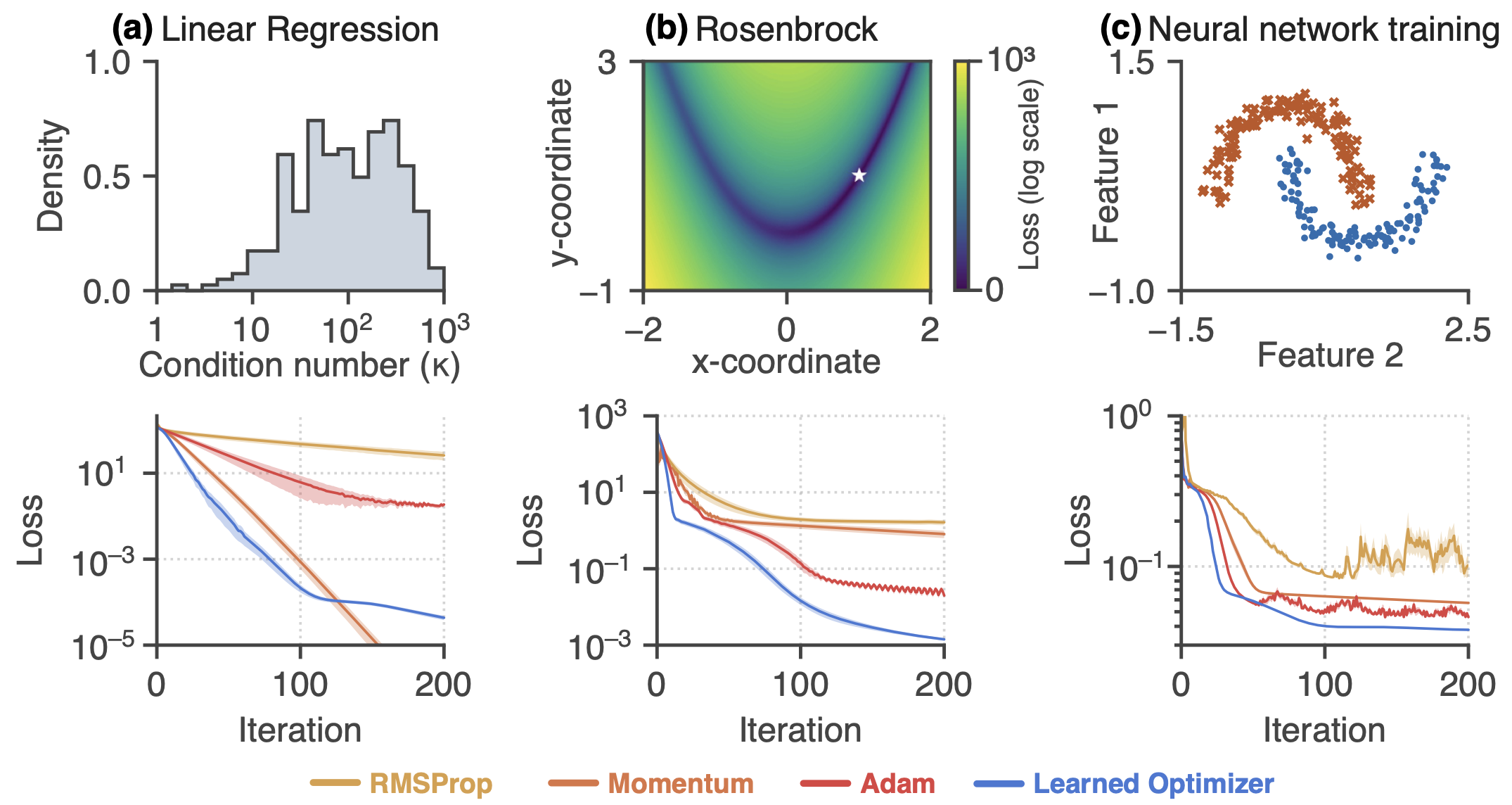Learned optimizers outperform well-tuned baselines on three different tasks. Upper row: Task schematics. Bottom row: Optimizer performance. [source]

The optimizers performance shown in the loss curves is the mean, standard deviation error over 128 random seeds. The learned optimizers outperform the three well-tuned optimizers on all three tasks.

## Momentum

In the experiment, each optimizer converges to a single global fixed point of the dynamic. The authors discovered that learned optimizers implement momentum using approximate linear dynamics. While they analyze the best performing learned optimizer, there is a learned optimizer on linear regression tasks with slightly worse performance but strongly resembled classical momentum. It recovered the optimal momentum parameters for that particular task distribution.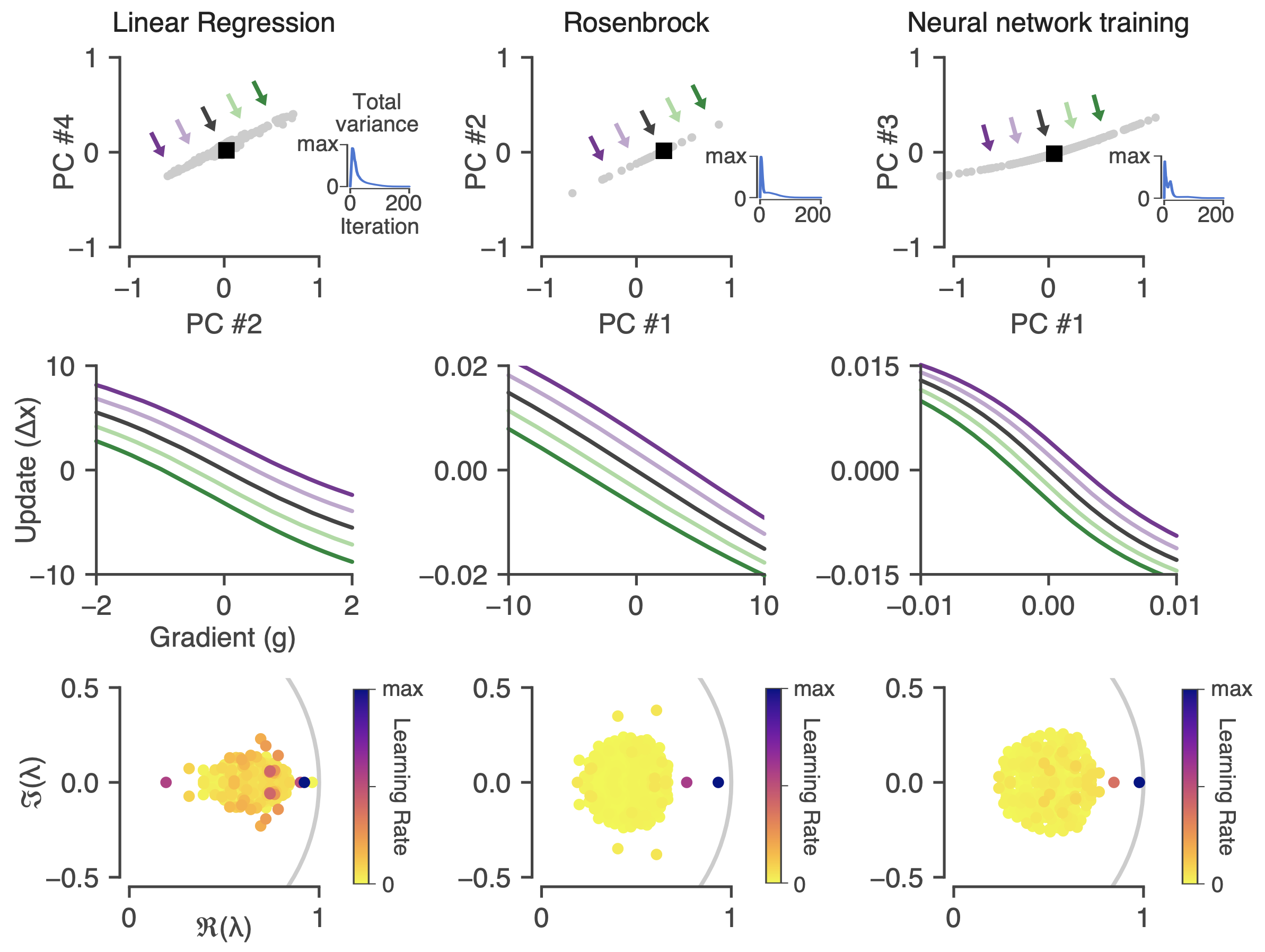Momentum in learned optimizers. Top row: Projection of the optimizer state around a convergence point. Middle row: Visualization of the update functions along the slow mode of the dynamics. Bottom row: Eigenvalues of the linearized optimizer dynamics at the convergence fixed point plotted in the complex plane. [source]

Learned optimizers also use saturating update functions as the gradient magnitude increases; this mimics a soft form of gradient clipping. In fact, the strength of the clipping effect is adaptive to the training task. For example, in the linear regression problem, the learned optimizer mainly stays within the update function’s linear region. In contrast, for the Rosenbrock problem, the learned optimizer utilizes a more saturating part of the update function.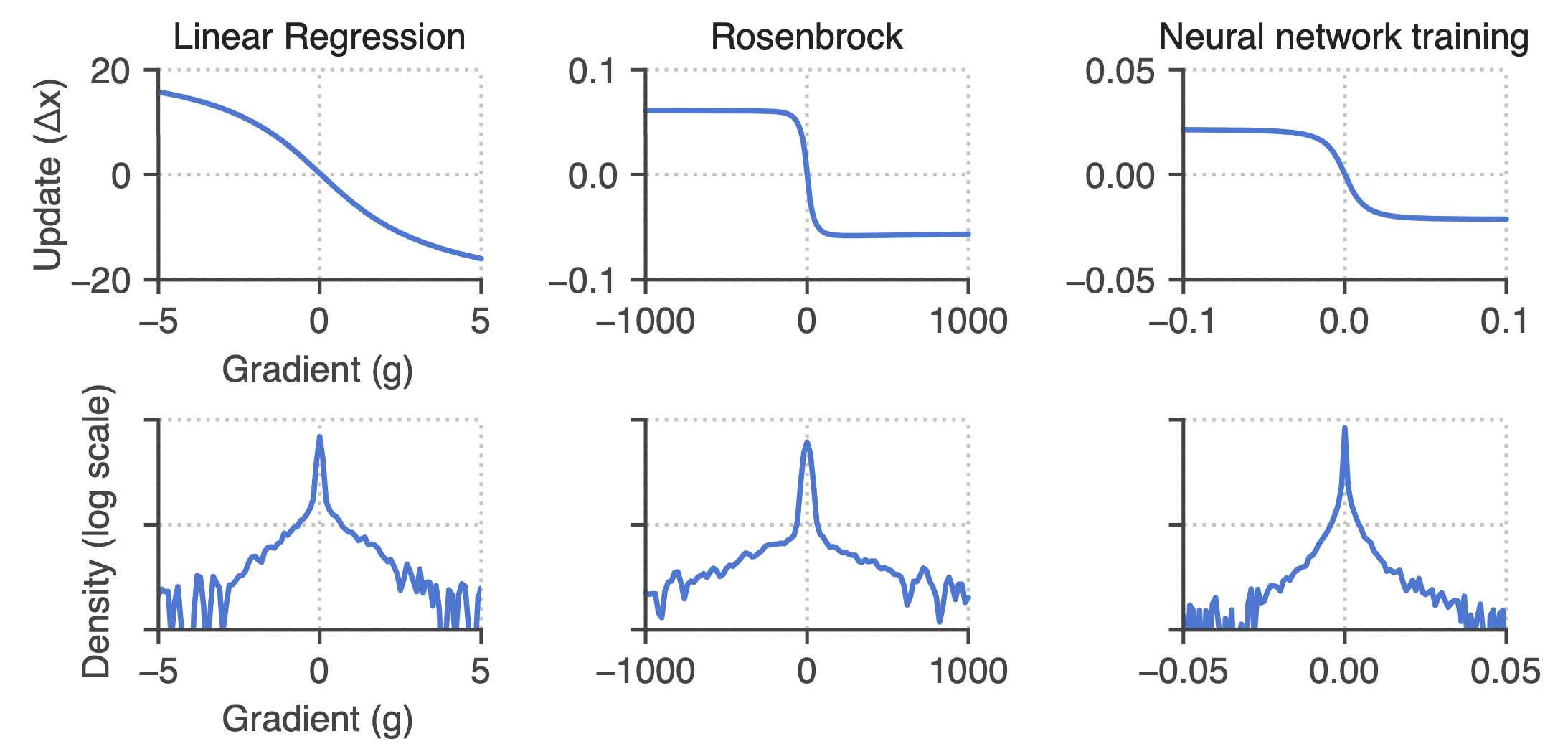Gradient clipping in a learned optimizer. Top row: The update function computed at the initial state saturates for large gradient magnitudes. The effect of this is similar to that of gradient clipping. Bottom row: The empirical density of encountered gradients for each task. [source]

## Learning rate schedules

We often tune the learning rate scheduler to decay the learning rate as the optimization progresses. The authors discovered that learned optimizers could implement a scheduler using autonomous dynamics. It encodes a particular trajectory as a function of the iteration as the system relaxes to the fixed point. From the figure below, in the linear regression experiment, the learned optimizer initially increases the learning rate of over 25 iterations, followed by a linear decay.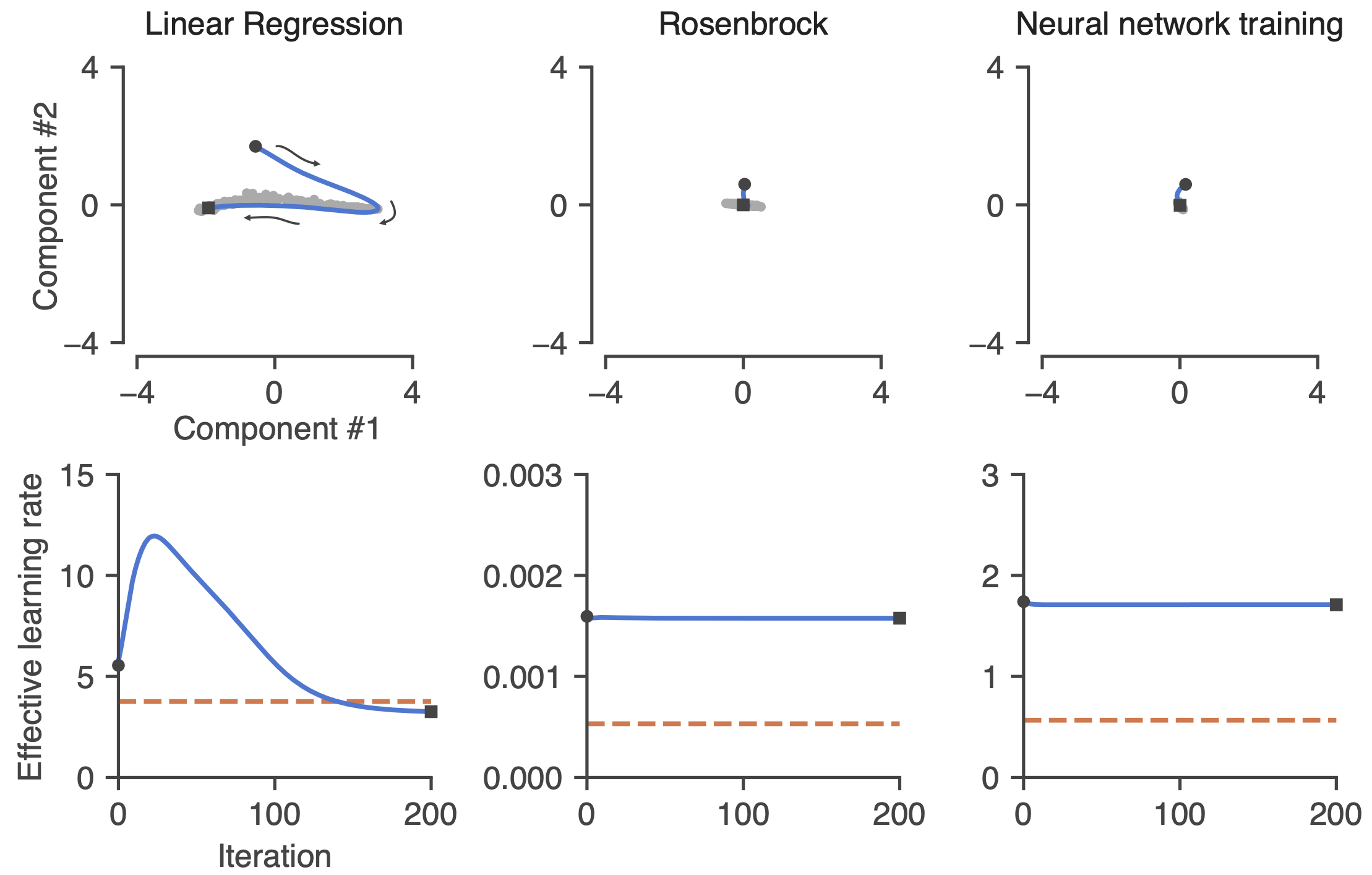Learning rate schedules mediated by autonomous dynamics. Top row: Low-dimensional projection of the dynamics of the learned optimizer in response to zero gradients (no input). These autonomous dynamics allow the system to learn a learning rate schedule. Bottom row: Effective learning rate as a function of iteration during the autonomous trajectories in the top row. [source]